# Time Series Forecasting with GRNN in R: the tsfgrnn Package

In this document the tsfgrnn package for time series forecasting using generalized regression neural networks (GRNN) is described. The package allows the user to build a GRNN model associated with a time series and use the model to predict the future values of the time series. It is possible to consult how the prediction has been done. Two different strategies for forecasting horizons greater than one are implemented. It is also possible to assess the forecast accuracy of the model.

# Introduction

A general regression neural network is a variant of an RBF network characterized by a fast single-pass learning. A GRNN consists of a hidden layer with RBF neurons. Normally, the hidden layer has so many neurons as training examples. The center of a neuron is its associated training example and so, its output gives a measure of the closeness of the input vector to the training example. Commonly, a neuron will use the multivariate Gaussian function:

$G(x, x_{i}) = \exp\left(- \frac{\| x - x_{i} \|^2}{2\sigma^2}\right)$

where $$x_{i}$$ and $$\sigma$$ are the center and the smoothing parameter respectively ($$x$$ is the input vector).

Given a training set consisting of $$n$$ training patterns (vectors $$\{x_{1}, x_{2}, \ldots x_{n}\}$$) and their associated $$n$$ targets, normally scalars ($$\{y_{1}, y_{2}, \ldots y_{n}\}$$), the GRNN output for an input pattern $$x$$ is computed in two steps. First, the hidden layer produces a set of weights representing the closeness of $$x$$ to the training patterns:

$w_{i} = \frac{\exp\left(- \frac{\| x - x_{i} \|^2}{2\sigma^2}\right)} {\sum_{j=1}^n \exp\left(- \frac{\| x - x_{j} \|^2}{2\sigma^2}\right)}$

Note that the weights decay with distance to the training pattern. The weights sum to one and represent the contribution of every training pattern to the final result. The GRNN output layer computes the output as follows:

$\hat{y} = \sum_{i=1}^n w_{i}y_{i}$

so a weighted average of the training targets is obtained, where the weights decay with distance to the training patterns. The smoothing parameter controls how many targets are important in the weighted average. When $$\sigma$$ is very large the result is close to the mean of the training targets because all of them have a similar weight. When $$\sigma$$ is small only the closest training targets to the input vector have significant weights.

# Time series forecasting with GRNN

In this section we describe how the tsfgrnn package can be used to predict the future values of a time series. The key function is grnn_forecasting that builds a model and uses the model to generate forecasts. Let us see an example:

library(tsfgrnn)
#> Warning: replacing previous import 'vctrs::data_frame' by 'tibble::data_frame'
pred <- grnn_forecasting(UKgas, h = 4)
#> Lag 3 Lag 1
#>     8    10
#>
#> $examples #> Lag3 Lag1 H1 H2 weight #> [1,] 1 3 4 5 0.000000e+00 #> [2,] 2 4 5 6 0.000000e+00 #> [3,] 3 5 6 7 5.358040e-190 #> [4,] 4 6 7 8 7.000777e-109 #> [5,] 5 7 8 9 8.619411e-46 #> [6,] 6 8 9 10 1.000000e+00 The output of grnn_weights shows that the GRNN is fed by the input (8, 10), the pattern associated with the forecast. We can see the weights of the different training examples. A weighted average of all the training targets is computed to generate the forecast. In this case, the last training pattern (6, 8) is the closest one to the input pattern and has practically all the weight (so the prediction will be very close to its target (9, 10)). If a large weight has been assigned to a training pattern is because the automatically selected smoothing parameter must be small. Let us check it with the summary function: summary(pred) #> #> Call: grnn_forecasting(timeS = 1:10, h = 2, lags = c(1, 3), msas = "MIMO", #> transform = "none") #> #> Multiple-Step Ahead Strategy: MIMO #> Sigma (smoothing parameter): 0.2195128 #> Autoregressive lags: 1 3 #> Type of training samples transformation: none #> Forecasting horizon: 2 #> Forecast: #> Time Series: #> Start = 11 #> End = 12 #> Frequency = 1 #>  9 10 It is possible to see a plot with the time series, the forecast, the input pattern and one of the training instances: library(ggplot2) plot_example(pred, 1)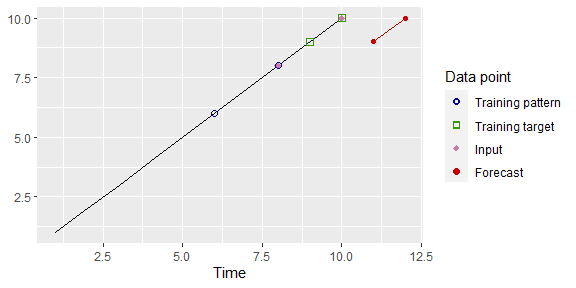In this case we have selected to see the closest training example (in blue and green) to the input pattern (in magenta), to select the fourth closest training example: plot_example(pred, 4)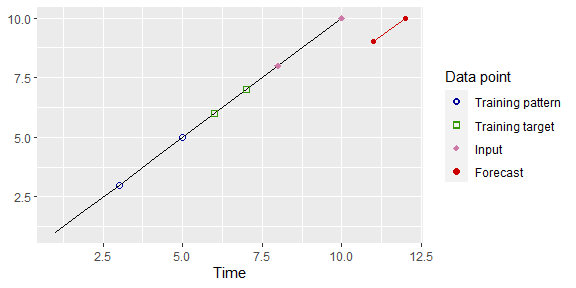## The recursive strategy In the recursive or iterative strategy a model that can only forecast one-step ahead is built. This model is applied iteratively to generate n-steps ahead forecasts. The recursive strategy is used by successful methodologies such as ARIMA or exponential smoothing. Let us see how this strategy works using the tsfgrnn package. First, we build a model and generate the forecasts for a given time series: predr <- grnn_forecasting(1:10, h = 2, lags = c(1, 3), msas = "recursive", transform = "none") predr$prediction
#> Time Series:
#> Start = 11
#> End = 12
#> Frequency = 1
#>  10 10
plot(predr)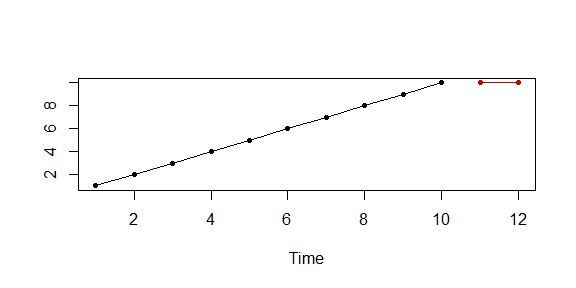Let us see the training examples of the model:

grnn_examples(predr)
#>      Lag3 Lag1 H1
#> [1,]    1    3  4
#> [2,]    2    4  5
#> [3,]    3    5  6
#> [4,]    4    6  7
#> [5,]    5    7  8
#> [6,]    6    8  9
#> [7,]    7    9 10

As can be seen, the length of the training targets is one, because the underlying model only predicts one-step ahead values. To see how, starting from a model that only predicts one-step ahead, the 2-steps ahead forecasts are generated we can use the plot_example function. Let us see first, the one-step ahead forecast:

plot_example(predr, position = 1, h = 1)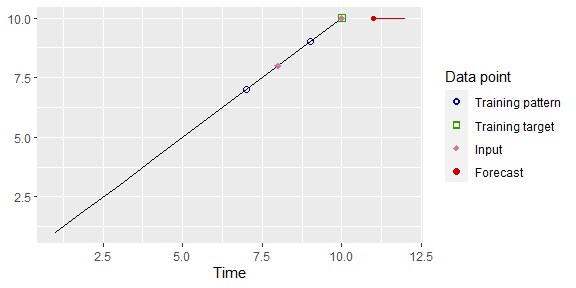In the function call, the parameter h indicates that we are interested in seeing the forecasting horizon 1 and the parameter position that we want to see the closest training example. In the forecast, in red, the horizon 1 is highlighted. The input vector used by the GRNN model are the lags 1 and 3 of the one-step ahead value, that is, the vector (8, 10), in magenta.

We can also see the weights of the training instances used in the prediction of the forecasting horizon 1:

grnn_weights(predr)[]
#> $input #> Lag 3 Lag 1 #> 8 10 #> #>$examples
#>      Lag3 Lag1 H1        weight
#> [1,]    1    3  4  0.000000e+00
#> [2,]    2    4  5  0.000000e+00
#> [3,]    3    5  6  0.000000e+00
#> [4,]    4    6  7 1.724617e-204
#> [5,]    5    7  8 2.119513e-109
#> [6,]    6    8  9  1.767415e-41
#> [7,]    7    9 10  1.000000e+00

Due to the fact that in a recursive prediction a one-step ahead model is used iteratively as many times as the length of the forecast horizon, the function grnn_weights returns a list with the different weights used by the model in the different predictions. As we are interested in the forecast for horizon 1 we consult the weights associated with that forecast. The training pattern with the highest weight is (7, 9), that was highlighted in the last plot.

Now, we plot information about how the forecast horizon 2 has been generated:

plot_example(predr, position = 1, h = 2)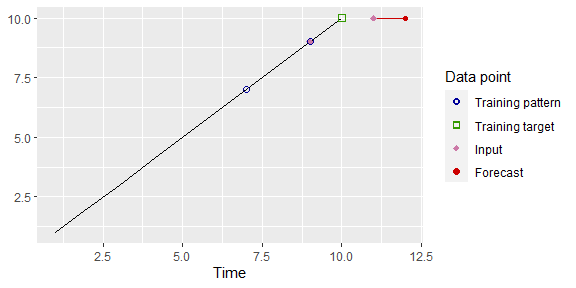In order to select the input vector associated with the forecasting horizon 2, the lags 1 and 3 are used. lag 3 (9) is obtained from the historical values of the time series. However, the time series cannot be used to obtain lag 1. In this situation, the recursive approach uses the predicted values. In this case, the lag 1 corresponds with the forecast for horizon 1, so the forecasted value (10) is used. This can also be checked with the grnn_weights function:

grnn_weights(predr)[]
#> $input #> Lag 3 Lag 1 #> 9 10 #> #>$examples
#>      Lag3 Lag1 H1        weight
#> [1,]    1    3  4  0.000000e+00
#> [2,]    2    4  5  0.000000e+00
#> [3,]    3    5  6  0.000000e+00
#> [4,]    4    6  7 3.048113e-245
#> [5,]    5    7  8 1.438120e-136
#> [6,]    6    8  9  4.603817e-55
#> [7,]    7    9 10  1.000000e+00

# Assessing the model

The function rolling_origin uses the rolling origin technique to assess the forecasting accuracy of a GRNN model. In order to use this function a GRNN model must have been built previously. We will use the artificial time series 1:20 to see how the function rolling_origin works:

pred <- grnn_forecasting(ts(1:20), h = 4, lags = 1:2)
ro <- rolling_origin(pred, h = 4)

The function rolling_origin uses the model returned by a grnn_forecasting call to apply rolling origin evaluation. The object returned by rolling_origin contains the results of the evaluation. For example, the test sets can be consulted as follows:

print(ro$test_sets) #> h=1 h=2 h=3 h=4 #> [1,] 17 18 19 20 #> [2,] 18 19 20 NA #> [3,] 19 20 NA NA #> [4,] 20 NA NA NA Every row of the matrix contains a different test set. The first row is a test set with the last h values of the time series, the second row a test set with the last h - 1 values of the time series and so on. Each test set has an associated training test with all the data in the time series preceding the test set. For every training set a GRNN model with the parameters associated with the original model is built and the test set is predicted. You can see the predictions as follows: print(ro$predictions)
#>      h=1 h=2 h=3 h=4
#> [1,]  17  18  19  20
#> [2,]  18  19  20  NA
#> [3,]  19  20  NA  NA
#> [4,]  20  NA  NA  NA

and also the errors in the predictions:

print(ro$errors) #> h=1 h=2 h=3 h=4 #> [1,] 0 0 0 0 #> [2,] 0 0 0 NA #> [3,] 0 0 NA NA #> [4,] 0 NA NA NA Several forecasting accuracy measures applied to all the errors in the different test sets can be consulted: ro$global_accu
#>  RMSE   MAE  MAPE SMAPE
#>     0     0     0     0

It is also possible to consult the forecasting accuracy measures for every forecasting horizon:

ro$h_accu #> h=1 h=2 h=3 h=4 #> RMSE 0 0 0 0 #> MAE 0 0 0 0 #> MAPE 0 0 0 0 #> SMAPE 0 0 0 0 Finally, a plot with the predictions for a given forecast horizon can be generated: plot(ro, h = 4)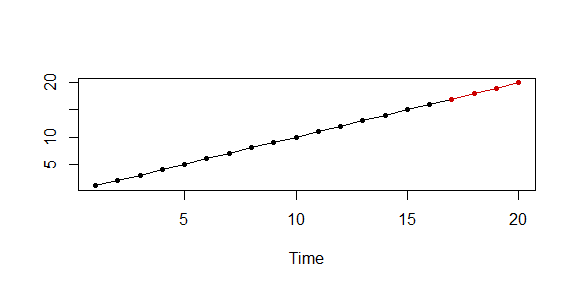The rolling origin technique is very time-consuming, if you want to get a faster assessment of the model you can disable this feature: ro <- rolling_origin(pred, h = 4, rolling = FALSE) print(ro$test_sets)
#>      h=1 h=2 h=3 h=4
#> [1,]  17  18  19  20
print(ro\$predictions)
#>      h=1 h=2 h=3 h=4
#> [1,]  17  18  19  20

In this case only one test and training set are used and therefore only one model is built.

# Preprocessing and model selection

In order to use a GRNN model for time series forecasting several parameters have to be selected. Furthermore, some preprocessing techniques can be applied. In the following subsections we give some information about this.

## Model selection

Apart from selecting the multi-step ahead strategy the user has to select the smoothing parameter and the autoregressive lags.

### The smoothing parameter

This parameter controls the level of smoothing of the training targets. When it is very large all the targets have a similar weight and therefore the forecast is close to the average of all the targets:

pred <- grnn_forecasting(USAccDeaths, h = 12, lags = 1:12, sigma = 100)
plot(pred)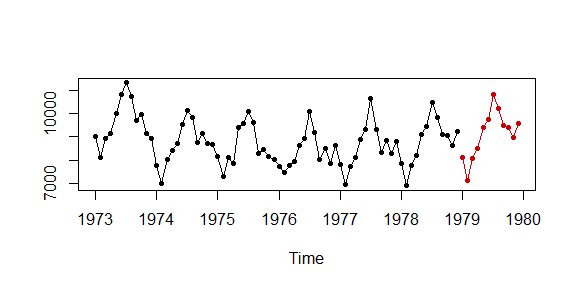On the contrary, when the smoothing parameter is very small just one or a few training targets have significant weights:

pred <- grnn_forecasting(USAccDeaths, h = 12, lags = 1:12, sigma = 0.05)
plot(pred)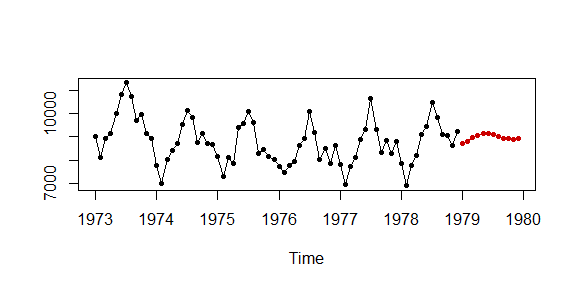As seen in previous examples, when this parameter is omitted in the function call it is automatically selected. The automatic selection is based on maximizing a forecast accuracy measure using the rolling origin evaluation.

### The autoregressive lags

The autoregressive lags set which lags are used to create the training instances. If you think that some lags can be important you should try to use them. You can also rely on the automatic selection. This is carried out according to the following criteria. The lags are 1:f, where f is the number of periods of the time series. For example, the lags for quarterly data are 1:4 and for monthly data 1:12. For time series in which the number of periods is one (for example, annual data) the lags with significant autocorrelation in the partial autocorrelation function (PACF) are selected. If no lag has a significant autocorrelation, then lags 1:5 are chosen.

As described above, currently it is possible to choose between the MIMO and recursive strategies. In our experience, the recursive strategy tends to produce better results.

## Transformations

Time series forecasting methodologies can take advantage of transforming the data. Next, we describe some of these techniques.

### Detrending

In the previous examples we have used time series, such as 1:10 or 1:20, with a constant trend. We have seen how GRNN is not capable of capturing these trends well. The reason is simple, GRNN predicts a weighted average of historical values of the time series, so that it cannot predict correctly values out of the range of the time series. In your time series has a trend we recommend using the parameter transform to transform the training samples. Use the value "additive"if the trend is additive or "multiplicative" for exponential time series:

set.seed(5)
timeS <- ts(1:10 + rnorm(10, 0, .2))
pred <- grnn_forecasting(timeS, h = 3, transform = "none")
plot(pred)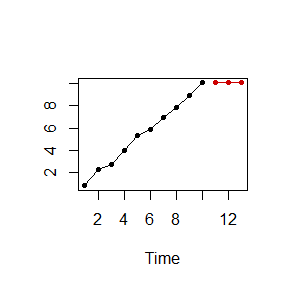pred2 <- grnn_forecasting(timeS, h = 3, transform = "additive")
plot(pred2)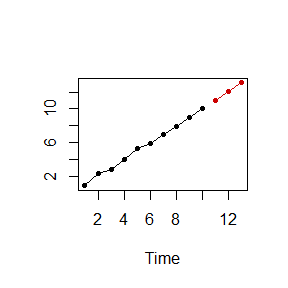### Seasonality

When a time series has a seasonal pattern it is important that the forecasting model captures this pattern. Currently, our package does not implement any strategy to deal with seasonal series. We think that GRNN is able to work directly with seasonal series as long as you set the right autoregressive lags. In the case of seasonal series we recommend to use lags 1:f where f is the length of the seasonal period.

# Acknowledgements

Funds: This work was partially supported by the project TIN2015-68854-R (FEDER Founds) of the Spanish Ministry of Economy and Competitiveness.